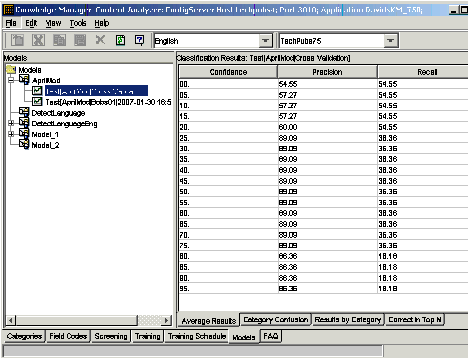Jump to: navigation, search

# Average Results Subtab

This topic describes part of the functionality of Genesys Content Analyzer.

The Average Results subtab, shown in the figure "Average Results Subtab," rates how well the model classifies, averaged across all categories.###### Models Tab: Average Results Subtab

The Confidence rating corresponds to the attribute of the same name that you set in the IRD objects Classify and Classification switch.

To understand Precision and Recall, consider several possible ways of looking at the performance of a model. If your model attempts to assign a certain number of items to a particular category X, you can make the following counts:

"a" = the number of items the model correctly assigns to X
"b" = the number of items the model incorrectly assigns to X
"c" = the number of items the model incorrectly rejects from X (that is, items that the model should assign to X but does not)

From these quantities, you can calculate the following performance measures:

• Precision = "a" /("a" + "b")
• Recall = "a" /("a" + "c")

Generally, for increasing precision you pay the price of decreasing recall. That is, the model assigns an item to a category only when it is very sure that the item belongs. But by insisting on being very sure, it runs the risk of rejecting items that really do belong in the category.
Here is another way to look at it. Suppose that at a confidence rating of 50, precision = 70 and recall = 80. Then, for text T and category C, the statements in the table "Example of Precision and Recall" hold.

### Example of Precision and Recall

If you know that

then you can infer that

this percent of the time

T belongs to C,

The model will classify T as C, with confidence of over 50%,

80%

The model classifies T as C, with confidence of over 50%,

T does belong to C,

70%

Use the Average Results ratings as an assessment of the overall quality of the model. See also Applying the Ratings.

This page was last modified on December 17, 2013, at 11:54.

## Feedback

Comment on this article:

blog comments powered by Disqus